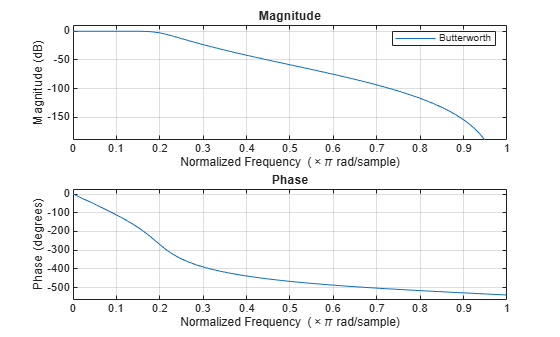Documentation

# stmcb

Compute linear model using Steiglitz-McBride iteration

## Syntax

```[b,a] = stmcb(h,nb,na) [b,a] = stmcb(y,x,nb,na) [b,a] = stmcb(h,nb,na,niter) [b,a] = stmcb(y,x,nb,na,niter) [b,a] = stmcb(h,nb,na,niter,ai) [b,a] = stmcb(y,x,nb,na,niter,ai) ```

## Description

Steiglitz-McBride iteration is an algorithm for finding an IIR filter with a prescribed time-domain impulse response. It has applications in both filter design and system identification (parametric modeling).

`[b,a] = stmcb(h,nb,na)` finds the coefficients `b` and `a` of the system b(z)/a(z) with approximate impulse response `h`, exactly `nb` zeros, and exactly `na` poles.

`[b,a] = stmcb(y,x,nb,na)` finds the system coefficients `b` and `a` of the system that, given `x` as input, has `y` as output. `x` and `y` must be the same length.

`[b,a] = stmcb(h,nb,na,niter)` and

`[b,a] = stmcb(y,x,nb,na,niter)` use `niter` iterations. The default for `niter` is 5.

`[b,a] = stmcb(h,nb,na,niter,ai)` and

`[b,a] = stmcb(y,x,nb,na,niter,ai)` use the vector `ai` as the initial estimate of the denominator coefficients. If `ai` is not specified, `stmcb` uses the output argument from `[b,ai] = ``prony``(h,0,na)` as the vector `ai`.

`stmcb` returns the IIR filter coefficients in length `nb+1` and `na+1` row vectors `b` and `a`. The filter coefficients are ordered in descending powers of z.

`$H\left(z\right)=\frac{B\left(z\right)}{A\left(z\right)}=\frac{b\left(1\right)+b\left(2\right){z}^{-1}+\cdots +b\left(nb+1\right){z}^{-nb}}{a\left(1\right)+a\left(2\right){z}^{-1}+\cdots +a\left(na+1\right){z}^{-na}}$`

## Examples

collapse all

Approximate the impulse response of an IIR filter with a system of lower order.

Specify a 6th-order Butterworth filter with normalized 3-dB frequency $0.2\pi$ rad/sample.

```d = designfilt('lowpassiir','FilterOrder',6, ... 'HalfPowerFrequency',0.2,'DesignMethod','butter');```

Use the Steiglitz-McBride iteration to approximate the filter with a 4th-order system.

```h = impz(d); [bb,aa] = stmcb(h,4,4);```

Plot the frequency responses of the two systems.

```hfvt = fvtool(d,bb,aa,'Analysis','freq'); legend(hfvt,'Butterworth','Steiglitz-McBride')```## Diagnostics

If `x` and `y` have different lengths, `stmcb` produces this error message:

```Input signal X and output signal Y must have the same length. ```

## Algorithms

`stmcb` attempts to minimize the squared error between the impulse response h of b(z)/a(z) and the input signal x.

`$\underset{a,b}{\mathrm{min}}\sum _{i=0}^{\infty }|x\left(i\right)-h\left(i\right){|}^{2}$`

`stmcb` iterates using two steps:

1. It prefilters `h` and `x` using 1/a(z).

2. It solves a system of linear equations for `b` and `a` using \.

`stmcb` repeats this process `niter` times. No checking is done to see if the `b` and `a` coefficients have converged in fewer than `niter` iterations.

## References

 Steiglitz, K., and L. E. McBride. “A Technique for the Identification of Linear Systems.” IEEE® Transactions on Automatic Control. Vol. AC-10, 1965, pp. 461–464.

 Ljung, Lennart. System Identification: Theory for the User. 2nd Edition. Upper Saddle River, NJ: Prentice Hall, 1999, p. 354.